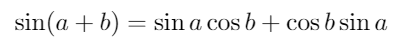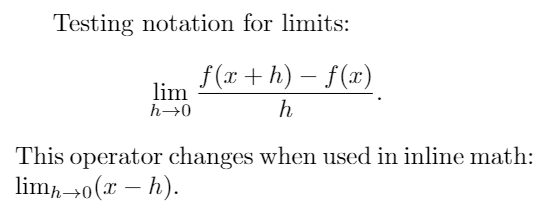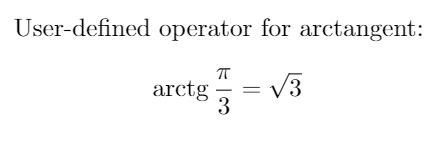Characters in mathematical mode are usually shown in italics, but sometimes certain function names require different formatting which can be accomplished by using operators defined in LaTeX.

# Introduction

Trigonometrical functions, logarithms, and others can be written in a document by means of some special commands, as demonstrated in the following example:

Examples of mathematical operators:
$\sin(a + b) = \sin a \cos b + \cos b \sin a .$


This example produces the following output:The commands will print the name of the function in upright (Roman) text instead of italics.

# Operators in different contexts

Some operators can take parameters that are handled in a special way, for instance, limits.

\documentclass{article}
\usepackage{amsmath}
\begin{document}
Testing notation for limits
$\lim_{h \to 0 } \frac{f(x+h)-f(x)}{h} .$
This operator changes when used alongside
text $$\lim_{h \to 0} (x-h)$$.
\end{document}


This example produces the following output:The package amsmath is needed for the macro \to; without this package, \rightarrow has to be used.

Notice how the limit declaration can include a subscript. See the reference guide for a complete list of available operators.

Some languages may add or change some commands, check the main page for language-specific articles.

If you need to add a personalized operator to be displayed in Roman font instead of italics use \DeclareMathOperator

\documentclass{article}
\usepackage{amsmath}
\DeclareMathOperator{\arctg}{arctg}
\begin{document}
User-defined operator for arctangent:
$\arctg \frac{\pi}{3} = \sqrt{3} .$
\end{document}


This example produces the following output:The command \DeclareMathOperator takes two parameters, the first one is the name of the new operator and the second one is the text to be displayed. For this command to work you have to import the package amsmath in the preamble with

\usepackage{amsmath}


The command can be slightly modified if you need that your defined operator uses subscripts, as the \lim operator, in such case use \DeclareMathOperator*.

# Reference guide

Complete list of mathematical operators

Operator Renders as
\cos $\cos$\csc $\csc$\exp $\exp$\ker $\ker$\limsup $\limsup$\min $\min$\sinh $\sinh$\arcsin $\arcsin$\cosh $\cosh$\deg $\deg$\gcd $\gcd$\lg $\lg$\ln $\ln$\Pr $\Pr$\sup sup
\arctan $\arctan$\cot cot
\det $\det$\hom $\hom$\lim lim
\log $\log$\sec sec
\tan $\tan$\arg $\arg$\coth $\coth$\dim $\dim$\liminf $\liminf$\max $\max$\sin $\sin$\tanh $\tanh$